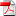# A review of optimal decision rule for investment in electricity generation

1
Août

RR-FiME-12-04

### Carmine De Franco, Peter Tankov, Xavier Warin

We develop algorithms for the numerical computation of the quadratic hedging strategy in incomplete markets modeled by pure jump Markov process. Using the Hamilton-Jacobi-Bellman approach, the value function of the quadratic hedging problem can be related to a triangular system of parabolic partial integro-differential equations (PIDE), which can be shown to possess unique smooth solutions in our setting. The first equation is non-linear, but does not depend on the pay-off of the option to hedge (the pure investment problem), while the other two equations are linear. We propose convergent finite difference schemes for the numerical solution of these PIDEs and illustrate our results with an application to electricity markets, where time-inhomogeneous pure jump Markov processes appear in a natural manner.

•rr-fime-12-04